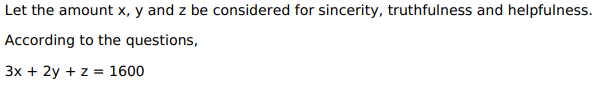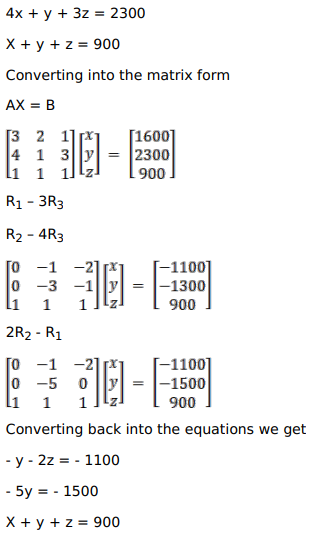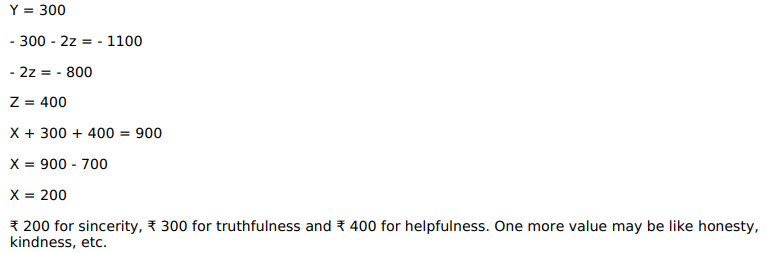# Solve this following

Question:

Two schools $A$ and $B$ want to award their selected students on the values of sincerity, truthfulness and helpfulness. The school $A$ wants to award $₹ x$ each, ₹ $y$ each and $₹$ each for the three respective values to 3,2 and 1 students respectively with total award money of ₹ 1,600 . School $B$ wants to spend $₹ 2,300$ to award its 4,1 and 3 students on the respective values (by giving the same award money to the three values as before). If the total amount of award for one prize on each value is $₹ 900$, using matrices, find the award money for each value. Apart from these three values, suggest one more value which should be considered for award.

HINT: By the given data, we have

$\left.\begin{array}{l}3 x+2 y+z=1600 \\ 4 x+y+3 z=2300 \\ x+y+z=900\end{array}\right\}$

Solution: